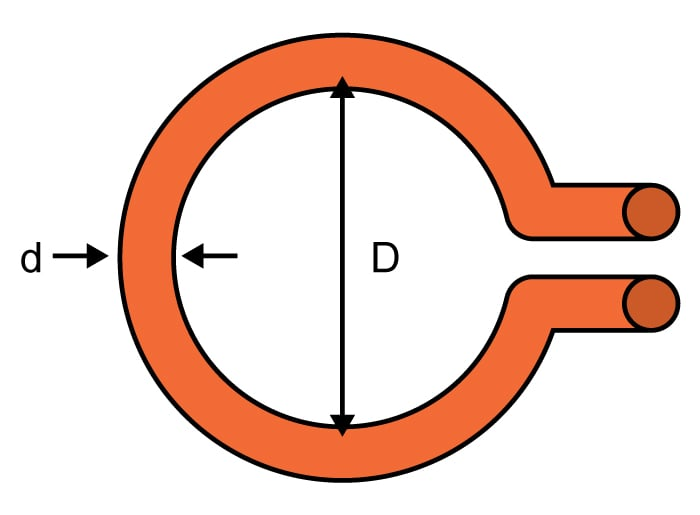# Wire Loop Inductance Calculator

## This calculator helps you compute the inductance of a wire loop.

H

### Overview

A loop of wire is a typical model for inductive materials. This calculator is designed to compute the inductance of a loop of wire given the diameter of the loop and the diameter of the wire used for the loop. To use this tool, just enter the diameter of the loop, the diameter of the wire, and the relative permeability. Since loops usually have air as the substrate, a relative permeability of 1 is often used in calculations. Note that it is possible to select the units of measurement for both the loop and the wire diameter.### Equations

$$L_{loop}\approx \mu _{o}\mu _{r}\left ( \frac{D}{2} \right )\cdot \left [ \ln\left ( \frac{8\cdot D}{d} \right )-2 \right ]$$

Where:

$$L_{loop}$$ = inductance of the wire loop in henries (H)

$$D$$ = loop diameter

$$d$$ = wire diameter

$$\mu_{r}$$ = relative permeability

$$\mu_{0}$$ = permeability of free space = 4π x 10-7

### Applications

Loop inductors are found in many areas in electrical and electronic engineering. Loops are often used for transmission and reception of radio signals (i.e. loop antenna). They are also used as metal detectors, as metal comes in contact with a magnetic loop, current is induced. A more modern use of loop inductors is in hearing aids. In this setup, one or more loops are used with a phase shift between them and placed near the area in which a hearing aid user would be present.